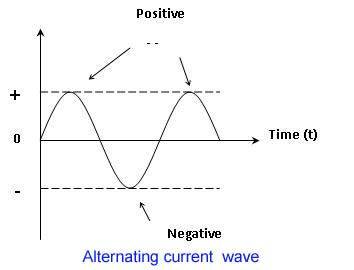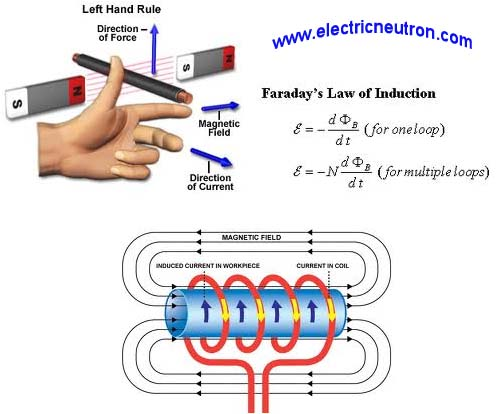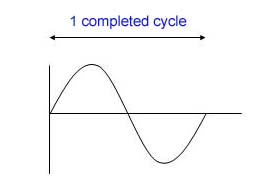# Alternating Current (AC)

### What is an alternating current?

Alternating current (ac) is changing the current flow.Changes in the flow of current in ac circuits because if the changes the polarity of the alternating voltage source alternately.

Current value will increase from zero to a maximum in the positive direction and then dropped to zero. Next, the current will be reduced to a maximum in the negative direction. Then, the current back up to zero.### Resources alternating current (A.C)

One source of alternating current generator is a machine that uses the principle of electromagnetic induction, where it converts mechanical energy to electrical energy

There are two ways to generate alternating voltage (ac) :-

1) Conduct mobile, stationary magnetic flux (live). (Conductors cut the flux)

2) The magnetic flux field moves, stationary conductor (live). (Flux cutting the conductor)

### How to generate alternating current (A.C)?

In a basic generator assuming a single conductor, a conductor is called (armature) and the end of this loop is connected to the ring of slip (slip rings).

Slip rings are insulated from each other and from the shaft where it mounted.carbon brush is connected to the ring lubricant to enable it to connect the conductor (armature) moves to the external circuit (terminal output).

Armature must be driven by mechanical force.Therefore, said generator that converts mechanical energy machine to electrical energy.

Energy conversion is based on the production of electromotive force (emf) when there is a flux cutting the conductor (armature) as specified by the Faraday law of electromagnetic induction.

The direction of current flow and the polarity of the emf depends on the direction of the magnetic field, the direction of rotation (movement) of the conductor. It can be determined by using the Right Hand Rule Fleming.### Definition of the cycle, frequency and period, a peak value and the amplitude

1) cycle

• For a complete cycle, the conductor of the generator rotate in a complete cycle. Here, the output voltage will have a change of sinus2) frequency

• Frequency of 1 Hertz (Hz) is defined as a cycle within one second.
• Frequency of ave form is usually obtained by inverse time period
3) period
• It mean time taken to complete one cycle of the wave
4) peak value and or amplitude
• defined as the high peak of a sine wave.
Root Mean Square ( RMS ) Voltage and ampere

1) RMS value = 0.707 Peak value
RMS value (root mean square) or effective value of sine wave shaped voltage is equal to 0707 at the peak value of the product
2) The average value = 0.637 Peak value
The average value of a sine wave for the positive half cycle is the peak to peak divided by the π
3) Peak current value
From formula :-
I rms = I max x 0.707
I max = I rms x 1.414

This site uses Akismet to reduce spam. Learn how your comment data is processed.